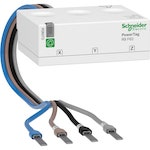# Schneider Electric A9MEM1570

Model A9MEM1570
Vendor Schneider Electric
Description PowerTag power sensor
Exposes power, power_apparent, power_phase_a, power_phase_b, power_phase_c, power_factor, energy, energy_phase_a, energy_phase_b, energy_phase_c, ac_frequency, voltage_phase_a, voltage_phase_b, voltage_phase_c, voltage_phase_ab, voltage_phase_bc, voltage_phase_ca, current_phase_a, current_phase_b, current_phase_c, linkquality
Picture## Exposes

### Power (numeric)

Instantaneous measured power. Value can be found in the published state on the `power` property. It’s not possible to read (`/get`) or write (`/set`) this value. The unit of this value is `W`.

### Power_apparent (numeric)

Instantaneous measured apparent power. Value can be found in the published state on the `power_apparent` property. It’s not possible to read (`/get`) or write (`/set`) this value. The unit of this value is `VA`.

### Power_phase_a (numeric)

Instantaneous measured power on phase A. Value can be found in the published state on the `power_phase_a` property. It’s not possible to read (`/get`) or write (`/set`) this value. The unit of this value is `W`.

### Power_phase_b (numeric)

Instantaneous measured power on phase B. Value can be found in the published state on the `power_phase_b` property. It’s not possible to read (`/get`) or write (`/set`) this value. The unit of this value is `W`.

### Power_phase_c (numeric)

Instantaneous measured power on phase C. Value can be found in the published state on the `power_phase_c` property. It’s not possible to read (`/get`) or write (`/set`) this value. The unit of this value is `W`.

### Power_factor (numeric)

Instantaneous measured power factor. Value can be found in the published state on the `power_factor` property. It’s not possible to read (`/get`) or write (`/set`) this value. The unit of this value is `%`.

### Energy (numeric)

Sum of consumed energy. Value can be found in the published state on the `energy` property. It’s not possible to read (`/get`) or write (`/set`) this value. The unit of this value is `kWh`.

### Energy_phase_a (numeric)

Sum of consumed energy on phase A. Value can be found in the published state on the `energy_phase_a` property. It’s not possible to read (`/get`) or write (`/set`) this value. The unit of this value is `kWh`.

### Energy_phase_b (numeric)

Sum of consumed energy on phase B. Value can be found in the published state on the `energy_phase_b` property. It’s not possible to read (`/get`) or write (`/set`) this value. The unit of this value is `kWh`.

### Energy_phase_c (numeric)

Sum of consumed energy on phase C. Value can be found in the published state on the `energy_phase_c` property. It’s not possible to read (`/get`) or write (`/set`) this value. The unit of this value is `kWh`.

### Ac_frequency (numeric)

Measured electrical AC frequency. Value can be found in the published state on the `ac_frequency` property. It’s not possible to read (`/get`) or write (`/set`) this value. The unit of this value is `Hz`.

### Voltage_phase_a (numeric)

Measured electrical potential value on phase A. Value can be found in the published state on the `voltage_phase_a` property. It’s not possible to read (`/get`) or write (`/set`) this value. The unit of this value is `V`.

### Voltage_phase_b (numeric)

Measured electrical potential value on phase B. Value can be found in the published state on the `voltage_phase_b` property. It’s not possible to read (`/get`) or write (`/set`) this value. The unit of this value is `V`.

### Voltage_phase_c (numeric)

Measured electrical potential value on phase C. Value can be found in the published state on the `voltage_phase_c` property. It’s not possible to read (`/get`) or write (`/set`) this value. The unit of this value is `V`.

### Voltage_phase_ab (numeric)

Measured electrical potential value between phase A and B. Value can be found in the published state on the `voltage_phase_ab` property. It’s not possible to read (`/get`) or write (`/set`) this value. The unit of this value is `V`.

### Voltage_phase_bc (numeric)

Measured electrical potential value between phase B and C. Value can be found in the published state on the `voltage_phase_bc` property. It’s not possible to read (`/get`) or write (`/set`) this value. The unit of this value is `V`.

### Voltage_phase_ca (numeric)

Measured electrical potential value between phase C and A. Value can be found in the published state on the `voltage_phase_ca` property. It’s not possible to read (`/get`) or write (`/set`) this value. The unit of this value is `V`.

### Current_phase_a (numeric)

Instantaneous measured electrical current on phase A. Value can be found in the published state on the `current_phase_a` property. It’s not possible to read (`/get`) or write (`/set`) this value. The unit of this value is `A`.

### Current_phase_b (numeric)

Instantaneous measured electrical current on phase B. Value can be found in the published state on the `current_phase_b` property. It’s not possible to read (`/get`) or write (`/set`) this value. The unit of this value is `A`.

### Current_phase_c (numeric)

Instantaneous measured electrical current on phase C. Value can be found in the published state on the `current_phase_c` property. It’s not possible to read (`/get`) or write (`/set`) this value. The unit of this value is `A`.

Link quality (signal strength). Value can be found in the published state on the `linkquality` property. It’s not possible to read (`/get`) or write (`/set`) this value. The minimal value is `0` and the maximum value is `255`. The unit of this value is `lqi`.